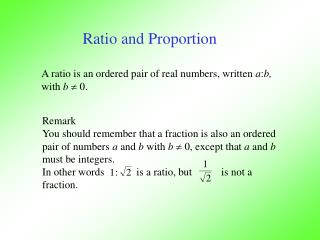DownloadDownload PresentationRatio and Proportion

# Ratio and Proportion

Download Presentation## Ratio and Proportion

- - - - - - - - - - - - - - - - - - - - - - - - - - - E N D - - - - - - - - - - - - - - - - - - - - - - - - - - -
##### Presentation Transcript

1. Ratio and Proportion A ratio is an ordered pair of real numbers, written a:b, with b 0. Remark You should remember that a fraction is also an ordered pair of numbers a and b with b0, except that a and b must be integers. In other words is a ratio, but is not a fraction.

2. Ratio and Proportion • Recall that a fraction is always used for part-to-whole comparison, but a ratio can be used for • part-to-part comparison • part-to-whole comparison • other comparisons such as length-to-width.

3. Ratio and Proportion Equality of ratios Two ratios a:b and c:d are equal if and only if a ×d = b ×c i.e. a : b = c : d if and only if inside×inside = outside×outside

4. Proportions A proportion is a statement that two given ratios are equal. • Practical examples: • If a cocktail recipe calls for 1 part of 7-up and 2 parts of orange juice, then you need to use the same ratio (no matter how much of cocktail wanted) in order to keep the taste consistent. • If you are mixing paint to paint your house, you need to keep the ratio (of color pigments to white paint) constant to ensure that the color will remain exactly the same.

5. Proportions • Practical examples: • If city tax rate is \$7.75 to every \$100 of purchase, then you have to use the same ratio no matter how much your purchase is (because it is the law). • If you want to enlarge a 4”×5” picture, then you should keep the same ratio in dimension to avoid distortion.

6. C 264Hz D 297Hz E 330Hz F 352Hz G 396Hz A 440Hz B 495Hz C’ 528Hz 15:16 8:9 9:10 8:9 15:16 9:10 8:9 Proportions Practical examples: 5. In music, it is the ratio of frequencies that determines the scale. The actual frequencies can vary a small amount. In C major scale, we have the following ratios (using the just-intonation scale)

7. Some situations where proportions are less understood. 1. Can Giants exist? Most of us like to watch movies and animations of giants or gigantic animals. But can we simply increase the size of a person (or an animal) and yet expect all other physical properties to remain the same?

8. Some situations where proportions less understood. 1. Can Giants exist? If we triple the size of a cube using the same material, the pressure (lb/ft2) on the bottom of the cube will become 3 times as big even though the foot print of the big cube is bigger.

9. Some situations where proportions are less understood. 1. Can Giants exist? Consequently, if a giant has the same body shape as a normal person and yet 3 times as high, his feet will take 3 times of the normal pressure, and his blood pressure has to be 3 times as high to pump blood from the feet back to his heart. This will put too much stress on the body and hence giants cannot live long if they ever exist.

10. Some situations where proportions are less understood. 2. Why babies can craw on their knees for a long time? On the other end of the spectrum, if a baby is only 1/3 of our height, then the pressure on its knees will be only 1/3 of ours, and such a small pressure will not cause pain in the knees.

11. Some situations where proportions are less understood. 3. Are babies just miniature adults? No, a baby’s body parts have proportions very different from those of adult’s. For instant, a baby has much smaller arm length/body length ratio than that of an adult.

12. Some situations where proportions are less understood. 3. Are babies just miniature adults? No, a baby’s body parts have proportions very different from those of adult’s. For instant, a baby has much smaller arm length/body length ratio than that of an adult.

13. Some situations where proportions are less understood. 4. How does the face change with age? The skull does not grow proportionally, hence the face does not simply enlarge when a child grows up.

14. Age Progression and Regression Photos JonBenet Ramsey Age Progressed from Age 6 to 16 Link

15. Can Giants exist? Tallest man ever lived. 8 feet 11.1 inches Tallest man alive, 2009. (8 ft 1 in)

16. Percent Math word Percent means per hundred, or part of 100. The symbol for percent is % Percent is always a part-to-whole comparison, and the whole is divided into 100 congruent pieces. Example 37% means 37/100, which means 0.37